Engineering ToolBox - Resources, Tools and Basic Information for Engineering and Design of Technical Applications!

# Radius of Gyration in Structural Engineering

## Radius of gyration describes the distribution of cross sectional area in columns around their centroidal axis.

In structural engineering the Radius of Gyration is used to describe the distribution of cross sectional area in a column around its centroidal axis.

The structural engineering radius of gyration can be expressed as

r = (I / A)1/2                                   (1)

where

r = radius of gyration (m, mm, ft, in...)

I = Area Moment Of Inertia       (m4, mm4, ft4, in4 ..)

A = cross sectional area (m2, mm2, ft2, in2...)

### Some typical Sections and their Radius of Gyration

#### Rectangle - with axis in center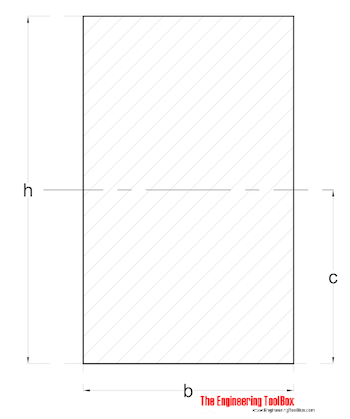Radius of Gyration for a rectangle with axis in center can be calculated as

rmax = 0.289 h                                (1)

where

rmax = max radius of gyration (strong axis moment of inertia)

#### Rectangle - with excentric axis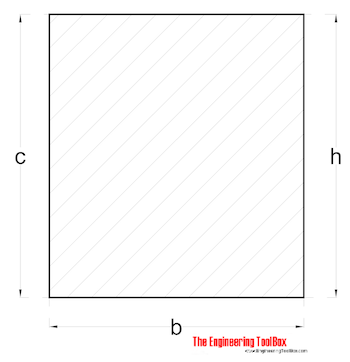Radius of Gyration for a rectangle with excentric axis can be calculated as

r = 0.577 h                                (2)

#### Rectangle - with tilted axis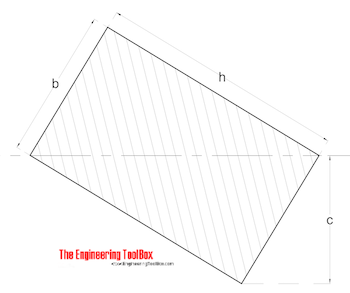Radius of Gyration for a rectangle with tilted axis can be calculated as

r = b h / (6 (b2 + h2))1/2                                (3)

#### Rectangle - with tilted axis II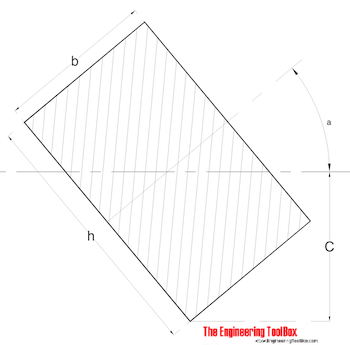Radius of Gyration for a rectangle with tilted axis can be calculated as

r = (((h2 + cos2a) + (b2 sin2a)) / 12)1/2                                (4)

#### Hollow Square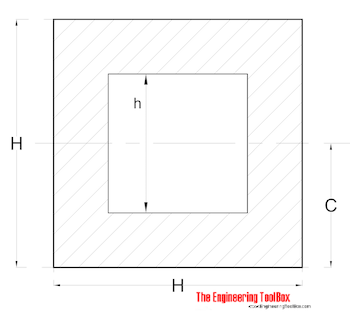Radius of Gyration for a hollow square can be calculated as

r = ((H2 + h2) / 12)1/2                                (5)

#### Hollow Square - with tilted axis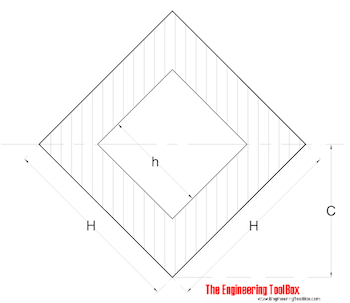Radius of Gyration for a hollow square with tilted axis can be calculated as

r = ((H2 + h2) / 12)1/2                                (6)

#### Equilateral Triangle with excentric axis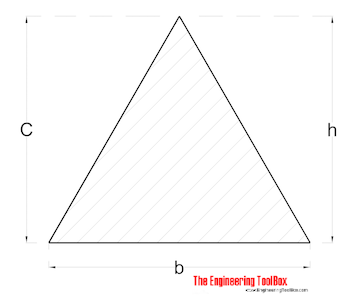Radius of Gyration for a equilateral triangle can be calculated as

r = h / (18)1/2                                (7)

#### Triangle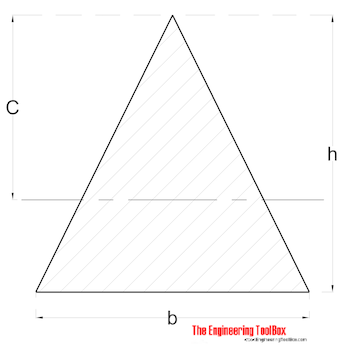Radius of Gyration for a equilateral triangle can be calculated as

r = h / (6)1/2                                (8)

## Related Topics

### • Beams and Columns

Deflection and stress, moment of inertia, section modulus and technical information of beams and columns.

### • Mechanics

Forces, acceleration, displacement, vectors, motion, momentum, energy of objects and more.

## Engineering ToolBox - SketchUp Extension - Online 3D modeling!

Add standard and customized parametric components - like flange beams, lumbers, piping, stairs and more - to your Sketchup model with the Engineering ToolBox - SketchUp Extension - enabled for use with the amazing, fun and free SketchUp Make and SketchUp Pro . Add the Engineering ToolBox extension to your SketchUp from the SketchUp Pro Sketchup Extension Warehouse!

Translate

## Privacy

We don't collect information from our users. Only emails and answers are saved in our archive. Cookies are only used in the browser to improve user experience.

Some of our calculators and applications let you save application data to your local computer. These applications will - due to browser restrictions - send data between your browser and our server. We don't save this data.